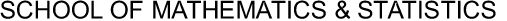University of SydneyApplied Mathematics SeminarUniversity of Sydney> Maths & Stats> Research> Applied Mathematics Seminar> Abstracts

### The Evaluation of European Compound Option Prices under Stochastic Volatility using Fourier Transform Techniques

Wednesday 24th March 14:05-14:55pm, New Law School Seminar 030 (Building F10).

This study focuses on European compound option pricing under stochastic volatility dynamics. Since a compound option is an option on an option, its value is not only sensitive to future movements of the underlying asset price, but also to future changes of volatility levels. Despite the existence of an analytical valuation formula for this type of option, the Black-Scholes model is not able to incorporate this sensitivity with respect to volatility in the option value. One approach to take the forward volatility into account is to value the option within a stochastic volatility model. Hence, the aim of this work is to develop a pricing procedure for compound options in stochastic volatility models, specifically focusing on the model of Heston (1993). In this case, the difficulty in the development of pricing methods compared to the Black-Scholes model lies in the additional dimension of uncertainty coming from the stochastic volatility.

Here, a numerical pricing algorithm is developed to solve this problem. It exploits that the representation of the compound option value can be divided into the difference of three probabilities under two different probability measures. These probabilities depend on three random variables (the future volatility and two future spot values) through a complex functional form. The joint distribution of these random variables under both measures can be uniquely determined by their joint characteristic function for each measure and therefore the probabilities can each be expressed as a multiple inverse Fourier transform. Solving the inverse Fourier transform with respect to volatility, the pricing problem is reduced to two dimensions and approximations of the three probabilities are obtained through a numerical fast Fourier transform technique. The resulting approximations are then compared with other numerical methods such as Monte Carlo simulations, showing promising results.